# Smooth morphism

(diff) ← Older revision | Latest revision (diff) | Newer revision → (diff)

of schemes

The concept of a family of non-singular algebraic varieties (cf. Algebraic variety) generalized to the case of schemes. In the classical case of a morphism of complex algebraic varieties this concept reduces to the concept of a regular mapping (a submersion) of complex manifolds. A finitely-represented (local) morphism of schemesis called a smooth morphism ifis a flat morphism and if for any point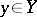the fibreis a smooth scheme (over the field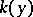). A schemeis called a smooth scheme over a scheme, or a smooth-scheme, if the structure morphismis a smooth morphism.

An example of a smooth-scheme is the affine space. A special case of the concept of a smooth morphism is that of an étale morphism. Conversely, any smooth morphismcan be locally factored with respect tointo a composition of an étale morphism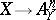and a projection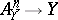.

A composite of smooth morphisms is again a smooth morphism; this is also true for any base change. A smooth morphism is distinguished by its differential property: A flat finitely-represented morphismis a smooth morphism if and only if the sheaf of relative differentials is a locally free sheaf of rank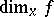at a point.

The concept of a smooth morphism is analogous to the concept of a Serre fibration in topology. E.g., a smooth morphism of complex algebraic varieties is a locally trivial differentiable fibration. In the general case the following analogue of the covering homotopy axiom is valid: For any affine scheme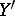, any closed subscheme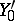of it which is definable by a nilpotent ideal and any morphism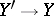, the canonical mapping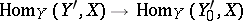is surjective.

Ifis a smooth morphism and if the local ringat the pointis regular (respectively, normal or reduced), then the local ring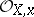of any pointwith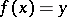will also have this property.

How to Cite This Entry:
Smooth morphism. Encyclopedia of Mathematics. URL: http://encyclopediaofmath.org/index.php?title=Smooth_morphism&oldid=15097
This article was adapted from an original article by V.I. DanilovI.V. Dolgachev (originator), which appeared in Encyclopedia of Mathematics - ISBN 1402006098. See original article